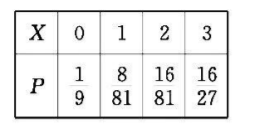2022 年 9 月 28 日晩, 中国女排在世锦赛小组赛第三轮比赛中, 又一次以 $3: 0$ 的比分酣畅淋漓地战胜了老对手日本女排, 冲上了热搜 榜第八位, 令国人振奋! 同学们, 你们知道排球比赛的规则和积分制 吗? 其规则是: 每场比赛采用 “ 5 局 3 胜制” (即有一支球队先胜 3 局即 获胜, 比赛结束). 比赛排名采用积分制, 积分规则如下: 比赛中, 以 3 : 0 或 $3: 1$ 取胜的球队积 3 分, 负队积 0 分; 以 $3: 2$ 取胜的球队积 2 分, 负队积 1 分. 已知甲、乙两队比赛, 甲队每局获胜的概率为 $\frac{2}{3}$.
(1) 如果甲、乙两队比赛 1 场, 求甲队的积分 $X$ 的概率分布列和数学 期望;
(2)如果甲、乙两队约定比赛 2 场, 求两队积分相等的概率.
【答案】 (1) 随机变量 $X$ 的所有可能取值为 $0,1,2,3$,
\begin{aligned} & P(X=0)=\left(\frac{1}{3}\right)^3+\mathrm{C}_3^1 \cdot \frac{2}{3} \cdot\left(\frac{1}{3}\right)^2 \cdot \frac{1}{3}=\frac{1}{9}, P(X=1)=\mathrm{C}_4^2 \cdot\left(\frac{2}{3}\right)^2 \cdot\left(\frac{1}{3}\right)^2 \cdot \frac{1}{3}=\frac{8}{81}, \\ & P(X=2)=\mathrm{C}_4^2 \cdot\left(\frac{2}{3}\right)^2 \cdot\left(\frac{1}{3}\right)^2 \cdot \frac{2}{3}=\frac{16}{81}, P(X=3)=\mathrm{C}_3^2 \cdot\left(\frac{2}{3}\right)^2 \cdot \frac{1}{3} \cdot \frac{2}{3}+\left(\frac{2}{3}\right)^3=\frac{16}{27}, \end{aligned}（2）记“甲、乙两队比赛两场后, 两从积分相等”为事件 $A$,

$=\frac{1}{9} \times \frac{16}{27}+\frac{8}{81} \times \frac{16}{81}+\frac{16}{81} \times \frac{8}{81}+\frac{16}{27} \times \frac{1}{9}=\frac{1120}{6561}$.